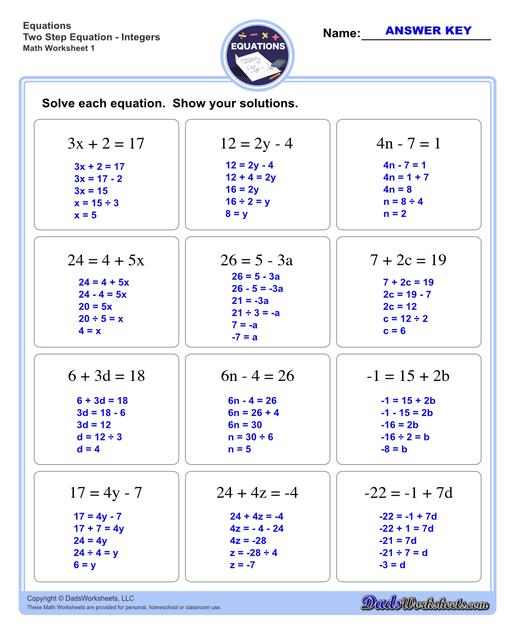PLEASE GO BACK AND USE THE BIG BLUE 'PRINT' BUTTON ON THE PAGE TO PRINT THE WORKSHEET CORRECTLY!Sorry for the trouble! The browser won't print the embedded worksheet PDF directly using the normal 'Print' command in the file menu, so you need to click the big 'Print' button to send just the worksheet and not the surrounding page to the printer.

# Math Worksheets: Two Step Equations : Two Step Equations Integers V1## Math Worksheets: Two Step Equations: Two Step Equations Integers V1

Property Value
DescriptionThe two step equations worksheets on this page include problems with integers, decimals and fractions for a variety of math operations. These beginning algebra worksheets are appropriate practice for 6th grade, 7th grade and 8th grade students. Full answer keys are included on the second page of each PDF file. Two Step Equations Integers V1
Resource TypeWorksheet